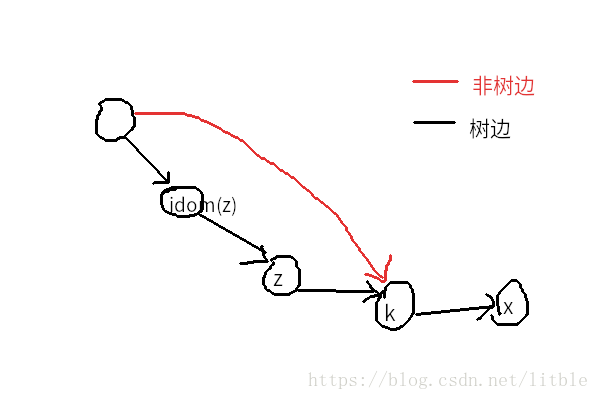前言

orz 一下这位大神

litble 特别菜，有错误请指出，谢谢。

How to build 支配树

DAG

DAG 的话，我们按照拓扑序从小到大进行，假设处理到点 $x$，则查一遍所有可达点 $x$的点 $y$，所有点 $y$一定被加入了支配树中，那么它们在支配树上的 LCA 就是 $x$在支配树上的父亲。

#include<bits/stdc++.h>
using namespace std;
#define RI register int
int q=0;char ch=' ';
while(ch<'0'||ch>'9') ch=getchar();
while(ch>='0'&&ch<='9') q=q*10+ch-'0',ch=getchar();
return q;
}
const int N=65540;
int n,top,js;
int f[N],du[N],p[N],st[N],ans[N],dep[N];
vector<int> g[N],rg[N],tr[N];
void topsort() {
for(RI i=1;i<=n;++i)
if(!du[i]) g.push_back(i),rg[i].push_back(0),++du[i];
top=1,st[top]=0;
while(top) {
int x=st[top];p[++js]=x,--top;
for(RI i=0;i<g[x].size();++i) {
--du[g[x][i]];
if(!du[g[x][i]]) st[++top]=g[x][i];
}
}
}
int lca(int x,int y) {
if(dep[x]<dep[y]) swap(x,y);
for(RI i=15;i>=0;--i) if(dep[f[x][i]]>=dep[y]) x=f[x][i];
if(x==y) return x;
for(RI i=15;i>=0;--i) if(f[x][i]!=f[y][i]) x=f[x][i],y=f[y][i];
return f[x];
}
void dfs(int x) {
ans[x]=1;
for(RI i=0;i<tr[x].size();++i)
dfs(tr[x][i]),ans[x]+=ans[tr[x][i]];
}
int main()
{
for(RI i=1;i<=n;++i) {
}
topsort();
for(RI i=2;i<=n+1;++i) {
int x=p[i],y=rg[x];
for(RI j=1;j<rg[x].size();++j) y=lca(y,rg[x][j]);
tr[y].push_back(x),dep[x]=dep[y]+1,f[x]=y;
for(RI j=1;j<=15;++j) f[x][j]=f[f[x][j-1]][j-1];
}
dfs(0);
for(RI i=1;i<=n;++i) printf("%d\n",ans[i]-1);
return 0;
}

一般有向图

半支配点

1. 假如在原图上删掉 $y$，$x$就不可达了，那么显然 $y$是 $x$在 dfs 树上的祖先。
2. 假若从 $y$的某个祖先出发，可以在不经过 $y$的情况下，走到一个 $dfn(y) < dfn(z) \leq dfn(x)$的点 $z$，$y$就是 $x$的支配点，反之不是。
3. 因为不能经过 $y$，所以从这个祖先走到 $z$的路径上经过的所有点的 $dfn$应该大于 $y$。
4. 假如这条路径上的所有点的 $dfn$都大于 $z$，则显然通过 $(semi(z),z)$可以保证新图上这个点依然能到 $z$。否则，这条路径要么经过一个 $dfn$小于等于 $x$大于 $y$的点（直接满足条件），要么全部经过 $dfn$大于 $x$的点（也就是 $x$的半支配点）
5. 所以，新图中的支配点关系与原图相同。

求半支配点

1. 考虑从 $semi(x)$到 $x$的那条只经过 $dfn$大于 $x$的点的路径上，$x$的前驱。若这个前驱是一个 $dfn$小于 $x$的点，那么只有可能从这个点出发是满足条件的。
2. 否则，这条路径上可能经过 $dfn$小于 $y$且大于 $x$的点（因为已经证明原图缩成 DAG 合法，所以不可能从 $dfn$大于 $y$的点走过来啦 QvQ），枚举这些点 $z$，它们的 $semi$就是满足条件的 $semi$。

从半支配点到支配点

1. 假设删掉 $idom(z)$，$x$依旧可达，则说明在 dfs 树上，$idom(z)$有一个祖先，可以走一条非树边（也就是通过 semi 连出来的边，图中红边）到达 $x$到 $idom(z)$中间的一个点 $k$。
2. 若 $z$不是 $k$的祖先，则删掉 $idom(z)$后 $z$仍可达，与支配点定义不符，所以 $z$是 $k$的祖先。
3. 那么因为 $z \in P$（我希望你还记得 $P$的定义），所以 $k \in P$。因为删除 $idom(z)$后 $semi(z)$不可达，所以 $dfn(semi(k)) \leq dfn(idom(z)) \leq dfn(semi(z))$，与我之前定义的 “$z$是 $P$中 $dfn(semi(z))$最小的点” 矛盾，所以该假设不可能成立。算法流程

（Tarjan 大神很喜欢 dfs 树和并查集啊）

Wraning: 数据出错，对于 $n$无法到的点，答案为 0，并且清空边集的时候，与 0 相连的边集也要清空（MDZZ 调了劳资一下午）

#include<bits/stdc++.h>
using namespace std;
#define RI register int
int q=0;char ch=' ';
while(ch<'0'||ch>'9') ch=getchar();
while(ch>='0'&&ch<='9') q=q*10+ch-'0',ch=getchar();
return q;
}
typedef long long LL;
const int N=50005,M=100005;
int n,m,tim;
int dfn[N],repos[N],mi[N],fa[N],f[N],semi[N],idom[N],ans[N];
struct graph{
int tot,h[N],ne[M],to[M];
void clear() {tot=0;for(RI i=0;i<=n;++i) h[i]=0;}//此题数据有误所以应从 i=0 开始清空
}g,rg,ng,tr;

void init() {
tim=0;g.clear(),rg.clear(),ng.clear(),tr.clear();
for(RI i=1;i<=n;++i)
repos[i]=dfn[i]=idom[i]=fa[i]=ans[i]=0,mi[i]=semi[i]=f[i]=i;
}
void tarjan(int x) {
dfn[x]=++tim,repos[tim]=x;
for(RI i=g.h[x];i;i=g.ne[i])
if(!dfn[g.to[i]]) fa[g.to[i]]=x,tarjan(g.to[i]);
}
int find(int x) {
if(x==f[x]) return x;
int tmp=f[x];f[x]=find(f[x]);
if(dfn[semi[mi[tmp]]]<dfn[semi[mi[x]]]) mi[x]=mi[tmp];
return f[x];
}
void dfs(int x,LL num) {
ans[x]=num+x;
for(RI i=tr.h[x];i;i=tr.ne[i]) dfs(tr.to[i],num+x);
}
void work() {
for(RI i=n;i>=2;--i) {
int x=repos[i],tmp=n;
for(RI j=rg.h[x];j;j=rg.ne[j]) {
if(!dfn[rg.to[j]]) continue;//此题数据有误
if(dfn[rg.to[j]]<dfn[x]) tmp=min(tmp,dfn[rg.to[j]]);
else find(rg.to[j]),tmp=min(tmp,dfn[semi[mi[rg.to[j]]]]);
}

x=repos[i-1];
for(RI j=ng.h[x];j;j=ng.ne[j]) {
int y=ng.to[j];find(y);
if(semi[mi[y]]==semi[y]) idom[y]=semi[y];
else idom[y]=mi[y];//此时 idom[mi[y]] 可能并未找到
}
}
for(RI i=2;i<=n;++i) {
int x=repos[i];
if(idom[x]!=semi[x]) idom[x]=idom[idom[x]];
}
dfs(n,0);
}
int main()
{
int x,y;
while(~scanf("%d%d",&n,&m)) {
init();
for(RI i=1;i<=m;++i)
tarjan(n);work();
for(RI i=1;i<n;++i) printf("%d ",ans[i]);
printf("%d\n",ans[n]);
}
return 0;
}

5 条评论孤月 · 2020年6月14日 4:58 下午

2020 年蒟蒻前来% 神仙，非常感谢这篇 blog。XZYQvQ · 2018年10月13日 11:01 上午